# Appendix B

## B1.0 Introduction

As discussed in Section 3.4, the spatial derivatives in the governing differential equations may be approximated using finite difference techniques. These techniques are the subject of this appendix. For a review of finite difference modeling, the reader is referred to Thomann and Mueller (1987).

We begin the discussion with a brief overview of the finite difference approximations required for the one-dimensional stream network. This overview is followed by a development of the equations for the individual segments or control-volumes that comprise the system. Two concluding sections discuss a special case of the approximations, boundary conditions.

## B2.0 Finite Difference Equations (FDEs)

Equation (1), the mass balance equation for the stream channel, contains several spatial derivatives that may be approximated via finite differences. In general, spatial derivatives and model parameters need to be defined at both the center of the stream segment and at the segment's interfaces. These locations are defined in Figure B1, where an arbitrary quantity, n, is shown. In the figure, the subscript `i' is used to represent the quantity at the center of the segment. Quantities at the upstream interface, where segment i-1 adjoins segment i, are indicated using the subscript `i-1,i'. In a similar fashion, the downstream interface is marked using the subscript `i,i+1'.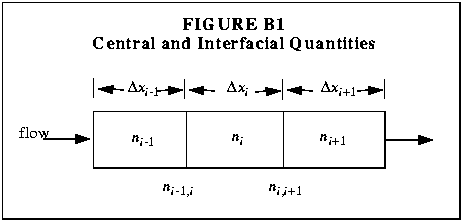### I. The Center of the Segment

The first task is to evaluate the spatial derivative, n/x, at the center of segment i. Using a centered difference, the spatial derivative of any variable is given by: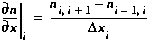(B1)

Note that Equation (B1) introduces the interfacial quantities, ni-1,i and ni,i+1. Since the mass balance equations were developed for the center of each segment, the interfacial quantities should be defined in terms of ni-1, ni and ni+1. To do this, we compute the interfacial quantities by interpolating linearly between the centers of the adjacent segments. This results in the following definitions: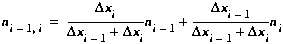(B2)(B3)

### II. The Segment Interfaces

We must also define the spatial derivatives at the upstream and downstream interfaces of the segment. These spatial derivative are defined as the change in n divided by the distance over which the change occurs. For the upstream interface, this gives: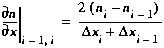(B4)

The same approach is used to evaluate the spatial derivative at the downstream boundary. This yields:(B5)

## B3.0 Interior Segments - FDEs

Using the foregoing definitions, it is now possible to approximate the spatial derivatives in Equation (1). Note that the first term on the right-hand side of Equation (1) is the `Advection' term while the second is the `Dispersion' term. These two quantities are now approximated for the interior segments of the stream network. Boundary segments, i.e. the first and last segments, represent special cases that are discussed in Sections B4.0 and B5.0.

Using definition (B1), the advective term may be approximated as: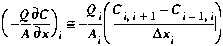(B6)

We now replace the interfacial concentrations (Ci,i+1 and Ci-1,i) with the definitions given by Equations (B2) and (B3). This substitution results in a centered difference approximation for C/x, given equal segment lengths: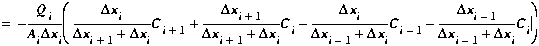(B7)

### II. Dispersion

Here again we use definition (B1) to define the spatial derivative: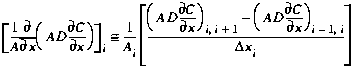(B8)

As the right side of Equation (B8) still contains spatial derivatives, we need the definitions given as Equations (B4) and (B5). Replacing the interfacial derivatives, we arrive at: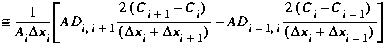(B9)

## B4.0 The First Segment - Upstream Boundary Condition

In the previous section, finite difference approximations for the spatial derivatives were developed. These approximations may be applied directly to all interior segments in the stream network. A problem arises, however, when we are dealing with the first segment, as all quantities involving the subscript i-1 are undefined. Equations (B7) and (B9) must be modified accordingly.

### I. Fixing the Upstream Boundary Condition

To effectively implement the finite difference equations for the first segment, an upstream boundary condition must be defined. This condition is shown in Figure B2, where the interfacial concentration at the upstream boundary is set to a user-supplied concentration, Cbc. This quantity is known as the upstream boundary condition.Given the definition of Cbc, Equation (B6) can be modified for use in the first segment:(B10)

As with the interior segments, replace the interfacial concentration (C1,2) using Equation (B3):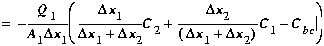(B11)

### III. Dispersion

Using the equation developed for the interior segments, the dispersive term is given by:(B12)

The first interfacial derivative (at 1,2) is defined as before using Equation (B5). The second term (at 0,1), requires an additional equation: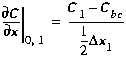(B13)

Note that the forward difference approximation shown above must be used in lieu of Equation (B4) to avoid introducing C0, the concentration in the non-existent segment zero. Equations (B5) and (B13) may now be used: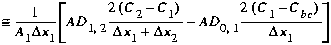(B14)

## B5.0 The Last Segment - Downstream Boundary Condition

The finite difference equations developed in Section B3.0 must also be modified to accommodate the last segment in the stream network. The required modifications to Equations (B7) and (B9) are shown below.

### I. Fixing the Downstream Boundary Condition

Application of Equations (B7) and (B9) to the last segment results in the introduction of an solute concentration, Ci+1. This concentration is undefined as segment i+1 does not exist. The last segment, i, and the fictitious segment, i+1, are shown in Figure B3.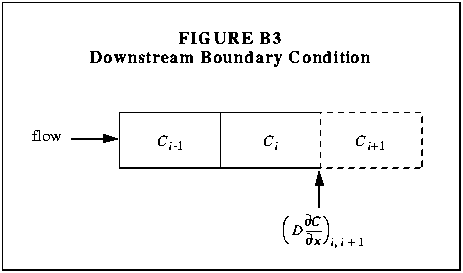To eliminate Ci+1 from the finite difference equations, the dispersive flux at the interface between segments i and i+1 is defined as in Equation (27):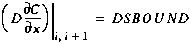(B15)

where DSBOUND is a user-supplied value for the dispersive flux. Note that if DSBOUND is set equal to zero, Equation (B15) is a zero gradient boundary condition (Arden and Astill, 1970).

Using the definition of the interfacial first derivative given by Equation (B5), Equation (B15) is solved for Ci+1, yielding: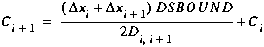(B16)

Equation (B16) is now used to eliminate Ci+1 from Equations (B7) and (B9).

Substituting Equation (B16) into (B7) and replacing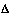xi+1 withxi, we arrive at the finite difference approximation of the advection term: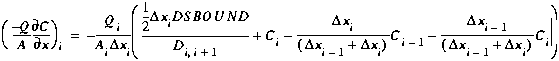(B17)
The finite difference approximation of the dispersion term is now developed by substituting Equation (B16) into (B9) and replacingxi+1 withxi: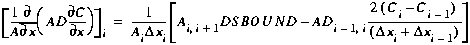(B18)